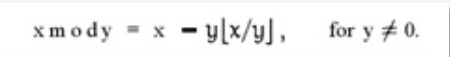# java基础-原码反码补码

## 1：原码反码补码，基础概念和计算方法

[+1]原 = 0000 0001

[-1]原  = 1000 0001

## 2：为什么要使用原码反码补码

1：利用反码和补码能将符号位带入计算（简化计算机门电路的设计，利用加法运算代替减法运算 1 - 1 = 1 + (-1) ）

// 利用反码进行计算

1 - 1 = 1 + (-1) = [0000 0001]原 + [1000 0001]原= [0000 0001]反 + [1111 1110]反 = [1111 1111]反 = [1000 0000]原 = -0

// 利用补码进行计算，可以解决反码中的 -0的问题

1-1 = 1 + (-1) = [0000 0001]原 + [1000 0001]原 = [0000 0001]补 + [1111 1111]补 = [0000 0000]补=[0000 0000]原

2：意外收获，利用补码进行计算 ，可以表示 -128（注意：-128没有反码和原码表示）

(-1) + (-127) = [1000 0001]原 + [1111 1111]原 = [1111 1111]补 + [1000 0001]补 = [1000 0000]补

因为机器使用补码, 所以对于编程中常用到的32位int类型, 可以表示范围是: [-2^31, 2^31 - 1] 因为第一位表示的是符号位.而使用补码表示时又可以多保存一个最小值.

## 3：理解一些现象

Integer.MAX_VALUE + 1 = Integer.MIN_VALUE
Integer.MIN_VALUE - 1 = Iegnter.MAX_VALUE 

## 4：原码反码补码深入理解

1：往回拨2h   6 -2 = 4

2：往前拨10h  (6 + 10)mod 12 = 4

3：往前拨 10 + 12 = 22h    (6 + 22) mod 12 = 4

### 4.1：同余的概念

eg：4 , 16 , 28 关于模12同余

4 mod 12 =4

16 mod 12 = 4

28 mod 12 = 4

### 4.2：负数取模eg:

-3 mod 2 = -3  - 2 * (-3/2)

= -3 - 2 * (-2)

= -3 + 4 = 1

### 4.3：开始证明

(-2) mod 12 = 10

10 mod 12 = 10      -2 和 10是同余的

(1)a ± c ≡ b ± d (mod m)

(2)a * c ≡ b * d (mod m)

现在我们为一个负数，找到了它的正数同余数，7 -2 ≡ 7 + 10 (mod 12)  即计算结果的余数相等

2-1=2+(-1) = [0000 0010]原 + [1000 0001]原= [0000 0010]反 + [1111 1110]反

(-1) mod 127 = 126

126 mod 127 = 126

(-1) ≡ 126 (mod 127)

2-1 ≡ 2+126 (mod 127)

2-1 与 2+126的余数结果是相同的! 而这个余数, 正式我们的期望的计算结果: 2-1=1

2-1=2+(-1) = [0000 0010]原 + [1000 0001]原 = [0000 0010]补 + [1111 1111]补

[0111 1111]原 = 127

(-1) mod 128 = 127

127 mod 128 = 127

2-1 ≡ 2+127 (mod 128)

09-14254102-104334
07-192963
08-28268
01-201万+
05-063033
03-2831
12-231343
04-053087
09-131万+
06-25100
07-0413万+
03-09339
03-221万+
01-19113
03-28822
03-31481
02-25440
06-3065
07-22135